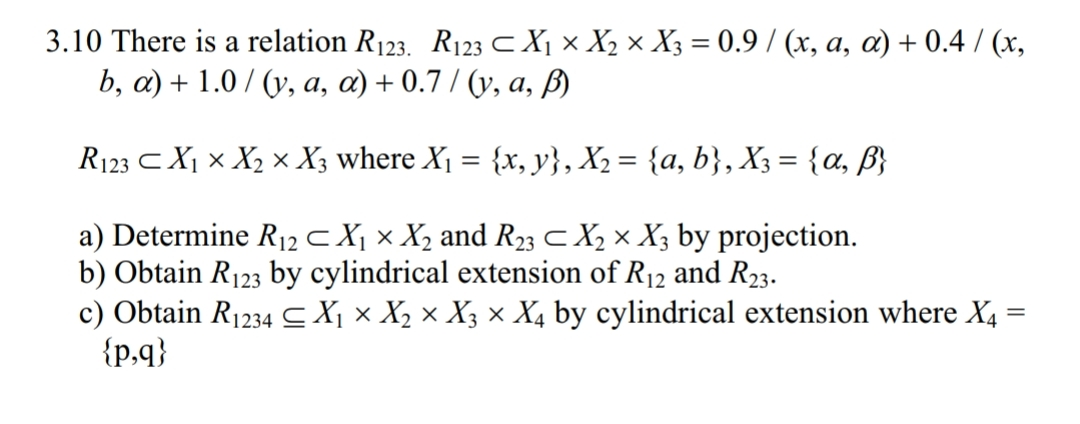3.10 There is a relation R123. R123X, x X2 × X3 = 0.9 / (x, a, α) + 0.4 / (x,a) Determine R12 X ×x, and R23 X2 × X3 by projection.b) Obtain R123 by cylindrical extension of R12 and R23.c) Obtain R1 234-X, x X2 x X3 x X; by cylindrical extension where X4lp,q)

Question

the book is "First Course on Fuzzy Theory and Applications"help_outlineImage Transcriptionclose3.10 There is a relation R123. R123 X, x X2 × X3 = 0.9 / (x, a, α) + 0.4 / (x, a) Determine R12 X ×x, and R23 X2 × X3 by projection. b) Obtain R123 by cylindrical extension of R12 and R23. c) Obtain R1 234-X, x X2 x X3 x X; by cylindrical extension where X4 lp,q) fullscreen
Step 1

Given: A relation 123 is a subset of

Step 2

(i) To determine:

Step 3

In order to project a fuzzy relati...

Want to see the full answer?

See Solution

Want to see this answer and more?

Our solutions are written by experts, many with advanced degrees, and available 24/7

See Solution
Tagged in

Math